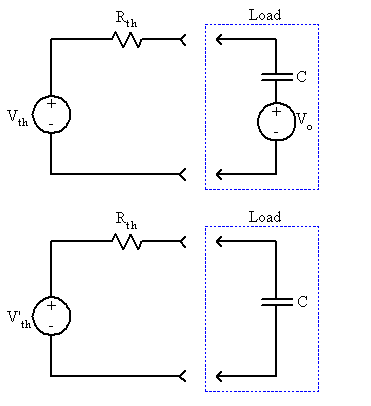# Transient response

## Homework Statement

http://s741.photobucket.com/user/pforpashya/media/studybitch/untitled_zps4cac3f38.png.html?filters[user]=137200878&filters[recent]=1&sort=1&o=0

## The Attempt at a Solution

Well I already got the solution and also verified answer in simulation.

My question is, while solving for t>0 current through capacitor I obtained using Thevenins eq.
while writing equation for vth i have to consider vc(0)=3v with the open circuited vth while applying mesh analysis.

Now if i try to do with nodal analysis and if i apply kcl at middle node do i have to consider the v(0)=3v in series with capacitor if no then please tell me why?

Last edited by a moderator:

gneill
Mentor

## Homework Statement

http://s741.photobucket.com/user/pforpashya/media/studybitch/untitled_zps4cac3f38.png.html?filters[user]=137200878&filters[recent]=1&sort=1&o=0

## The Attempt at a Solution

Well I already got the solution and also verified answer in simulation.

My question is, while solving for t>0 current through capacitor I obtained using Thevenins eq.
while writing equation for vth i have to consider vc(0)=3v with the open circuited vth while applying mesh analysis.

Now if i try to do with nodal analysis and if i apply kcl at middle node do i have to consider the v(0)=3v in series with capacitor if no then please tell me why?
Hi pforpashya. Welcome to Physics Forums.

I'm not sure that I understand your question accurately. I believe that you wish to know when and why to include the initial capacitor voltage in the analysis, is that correct?

For the first step in your analysis you determined the potential on the capacitor at time t = 0 (just before the switch K is closed). That becomes the initial potential on the capacitor for the next steps in the analysis. Since you're only looking to find the capacitor current you don't really care where the initial capacitor voltage ends up in the modelling process.

If you choose to use a Thevenin equivalent model for the analysis, then you can either treat the capacitor and its initial voltage as the load that is separated from the circuit when you build the model, or you can take the uncharged capacitor as the load and leave its initial voltage with the rest of the circuit when you create the Thevenin model. So the choices are:In the top circuit, the initial capacitor voltage Vo remains with the load and the Thevenin voltage Vth depends only on the 5V source in your circuit. In the bottom circuit, Vo has been folded into the Thevenin model, so V'th depends upon both the 5V source and Vo.

#### Attachments

i have to verify answer with Nodal analysis. When I am applying nodal analysis in the middle node what will be the equation of current flowing through capacitor?

if i consider node to be v1 then current through capacitor is 0.5 d/dt(v1-3)? or 0.5d/dt (V1)?...the battery present due to initial condition, is it becoming redundant when i apply kcl?

In same way is it true for inductor, that current initially flowing through inductor not considered when applying kvl to that loop,just it is utilize to calculate k?

Last edited by a moderator:
gneill
Mentor